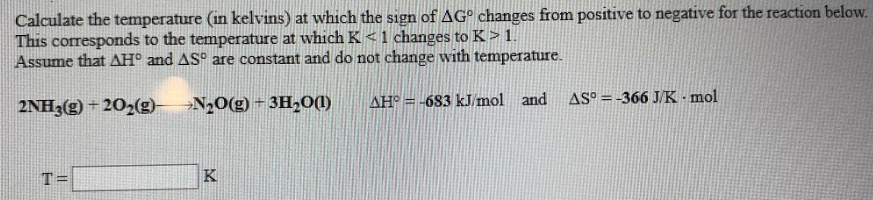# Calculate the temperature (in kelvins) at which the sign of ΔG° changes from positive to negative for the reaction below. This corresponds to the temperature at which K < 1 changes to K > 1. Assume that ΔH° and ΔS° are constant and do not change with temperature. 2NH3(g) + 2O2(g) → N2O(g) + 3H2O(l) ΔH° = -683 kJ/mol and ΔS° = -366 J/K • mol